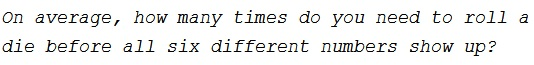# Waiting for All Six Outcomes

### Problem### Solution 1

Waiting time for an event that occurs with probability $p$ is known to be $\displaystyle \frac{1}{p}.$

Assume we already saw $k\lt 6$ numbers. The probability of seeing one of the remaining $6-k$ numbers after that is $\displaystyle \frac{6-k}{6},$ making the waiting time equal $\displaystyle \frac{6}{6-k}.$

Naturally, we start counting from $k=0;$ and the first roll of a die is always a success: one of the numbers shows up. Knowing that the expectation is additive, the expected number of rolls until all six numbers shows up is

$\displaystyle \sum_{k=0}^5\frac{6}{6-k}=6\left(\frac{1}{6}+\frac{1}{5}+\frac{1}{4}+\frac{1}{3}+\frac{1}{2}+\frac{1}{1}\right)\approx 14.7.$

### Solution 2

Let $t(n)$ be the number of throws till all outcomes, starting from $n$ distinct outcomes. On the next throw, with the probability $\displaystyle \frac{n}{6}$ we do not get an outcome that we had not before, and with probability $\displaystyle \frac{6-n}{6}$ we do get a new outcome. Solve

$\displaystyle t(n) = 1 + \frac{n}{6}\cdot t(n) + \frac{6-n}{6}\cdot t(n+1)$

for $t(0)$ with $t(6)=0.$ Explicitly, $\displaystyle t(n)-t(n+1)=\frac{6}{6-n}.$ Thus

\displaystyle \begin{align} t(5)-t(6)&=\frac{6}{1}\\ t(4)-t(5)&=\frac{6}{2}\\ t(3)-t(4)&=\frac{6}{3}\\ t(2)-t(3)&=\frac{6}{4}\\ t(1)-t(2)&=\frac{6}{5}\\ t(0)-t(1)&=\frac{6}{6}\\ \end{align}

Summing up, $\displaystyle t(0)=\sum_{n=0}^5\frac{6}{6-n}\approx 14.7.$

### Acknowledgment

This is a problem from P. Winkler's Mathematical Puzzles: A Connoisseur's Collection, (A K Peters, 2004, pp 35,37). The problem is popularly known as the coupon problem: drawing from an urn with replacement until all initial items have been drawn at least once.

Solution 2 is by David Koops.